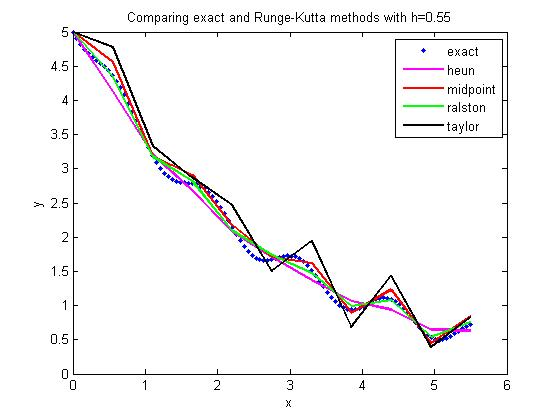## Comparing Runge-Kutta 2nd order methods

Many a times, students ask me

Which of the Runge-Kutta 2nd order methods gives the most accurate answer to solving a first order ODE?

dy/dx=f(x,y), y(0)=y0

There is no direct answer, although Ralston’s method gives a minimum bound for the truncation error (Ralston, A., Runge-Kutta Methods with Minimum Error Bounds, Match. Compu., Vol 16, page 431, 1962).

They also ask me if using the first three terms of the Taylor series would give a more accurate answer if we calculate$f^{\prime}(x,y)$ symbolically.

The equations for the four methods are given belowHere is the comparison graph for

dy/dx=sin(5*x)-0.4*y, y(0)=5

with

step size of h=1.1and

step size of h=0.55This post is brought to you by Holistic Numerical Methods: Numerical Methods for the STEM undergraduate at http://numericalmethods.eng.usf.edu